TM as Transducers

# TM as Transducers - Theory of Computation - Computer Science Engineering (CSE)

• Part III. TM as Transducers

TM as Transducers
In all the above TMs, TM either accepts or rejects a string. That is, TM acts as a language acceptor.
- A TM can function as a transducer. That is, a string is given as input to TM, it produces some output. We can view a Turing machine as a transducer.

Input to the computation is a set of symbols on the tape.
At the end of computation, whatever remains on the tape is the output.

- A TM can be viewed as a transducer for the implementation of function f defined as,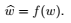- A function, f is said to be computable or Turing computable, if there exists a TM M =     (Q,∑, Γ, δ, q0, #, qf ) such that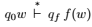, where w is in the domain of f. We can see that all common mathematical functions are turing computable.

This means, basic operations such as addition, subtraction, multiplication, division can be performed on it. This means that a TM is an abstract model of our modern computer system.

Example 1:
Design a Turing machine that computes the following function, f(m, n) = m + n. A positive integer on a Turing tape can be represented by an equal number of 0s.
For example,
integer 5 is represented as 00000, and
integer 8 is represented as 00000000.
In the tape two integers are separated using the symbol, \$.

Let us assume that m=3 and n=5.
Then the input tape of the Turing machine will be,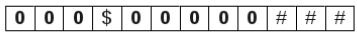After addition, tape will contain the results of addition as shown below: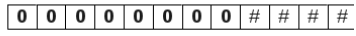TM for addition works as follows:
- Head reads the 0s of the first number, m and reaches the separator symbol, \$. Symbol, \$ is replaced with 0 and head moves towards right.
- It continues to move towards right till the second number, n is passed over and # is reached.
- At #, it turns left and replaces rightmost 0 with #.
- Now the tape contains the sum of m and n, and TM halts.
- Conversion of the separator symbol, \$ to 0, helps TM to make the single number on the tape. The conversion of symbol, 0 to # removes the additional 0 that was generated from the conversion of \$ to 0.

Transition table for the TM is shown below: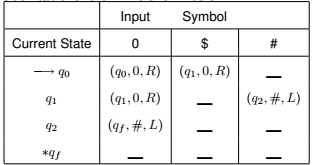Above table is describes as follows:
1. Initially, TM is in state q0. Head reads the first symbol, 0 and moves towards right without changing the state.
2. Head continues to move towards right till the separator symbol, \$ is reached. It replaces \$ with 0, changes state to q1, and continues to move towards right.
3. Head continues to move towards right and passes over the second number to reach #.
4. On reaching the #, head changes state to q2 and turns left.
5. In state q2, it replaces symbol 0 with #, and changes state to qf .
6. In the final state, TM halts to indicate the completion of the process.

Addition of 3 and 5 is shown below: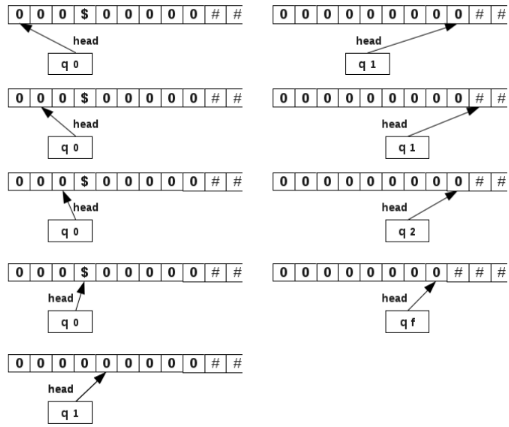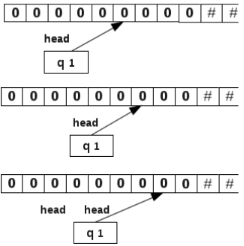Example 2:
Design a Turing machine that can multiply two numbers.
f(m, n) = m x n.

Let m=2, n=4
Tape contains two integers, both containing a termination symbol \$, at the end as shown below: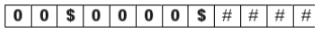After the multiplication operation, tape contains the result as shown below: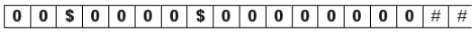TM for multiplication works as follows:
- Leftmost 0 is replaced with symbol x and head moves towards right.
- Head moves towards right till the remaining 0s are passed over and the separator symbol \$ is reached. It crosses \$ and reaches the leftmost 0 of the number n. It replaces this 0 with y and moves towards right.
- Head moves towards right and reaches and crosses the second separator symbol, \$.
- After crossing \$, it replaces # with 0.
- Now head turns towards left and replaces second leftmost 0 of n to y.
- Head then turns right and keeps moving towards right till a # is reached. It replaces # with 0 and turns towards left.
- This process is repeated till all the 0’s in the number n are converted to y and their copy is made after the second \$. This completes one cycle of copying.

- After one cycle of copying, head turns left and converts all y’s to 0. It moves towards left and converts the second leftmost 0 of m to x and makes a copy of n after the second \$.
- The cycle of copying n after the second \$ is repeated m times and the number of such cycles is tracked with the help of the number of x symbols in the number m. With m cycles of copying n after the second \$, tape contains mxn numbers of 0s, which is the product.

Transition table for the TM is shown below: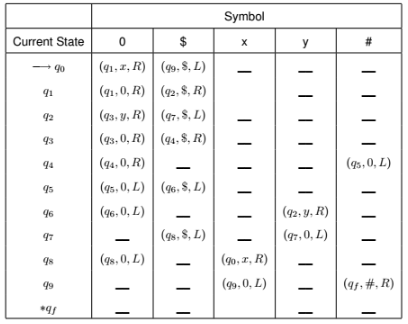Example 3:
Design a TM that copies strings of 1’s.

For this problem, let the given string is 11 as shown below: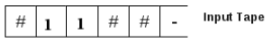Note that we store a # before the string in the tape.
The output from TM should be as follows: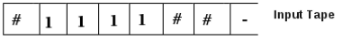Following is the transition table for this TM: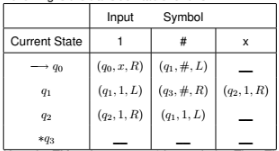Here the TM replaces every 1 by symbol x. Then TM replaces rightmost x by 1. It goes to the right end of the string and writes a 1 there. Thus TM has added a 1 for the rightmost 1 in the input string. This process is repeated.
TM reaches q1 after replacing all 1’s by x’s and reading the # symbol at the end of the input string. After replacing x by 1, TM reaches q2. TM reaches q3 at the end of the process and halts.
If the string is 11, we get 1111 at the end of computation.
If the string is 111, we get 111111 at the end of computation.

Consider the processing of the string 111: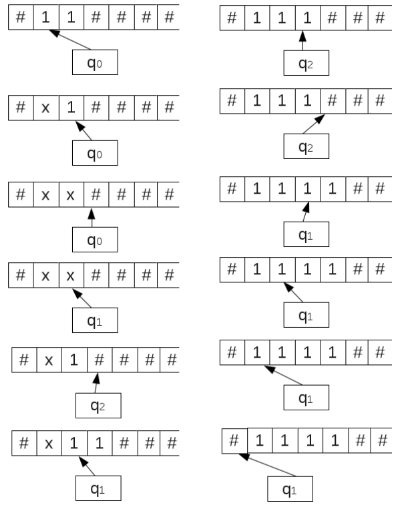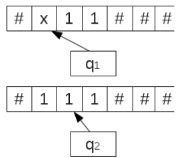TM as a Computer
Thus a TM can perform the basic operations such as addition, multiplication, subtraction and division. That means it can act as a computer. TM is a simple mathematical model of a computer. TM can do everything a computer can do. If TM cannot solve certain problems, then these problems are beyond the theoretical limits of computation.

Exercises:
1. Design a Turing machine that computes m - n where m and n are positive integers and m>n.
2. Design a TM to divide m by 3 and to compute the quotient and the remainder.

The document TM as Transducers | Theory of Computation - Computer Science Engineering (CSE) is a part of the Computer Science Engineering (CSE) Course Theory of Computation.
All you need of Computer Science Engineering (CSE) at this link: Computer Science Engineering (CSE)

## Theory of Computation

18 videos|56 docs|44 tests

## FAQs on TM as Transducers - Theory of Computation - Computer Science Engineering (CSE)

 1. What is a transducer in computer science engineering?Ans. A transducer in computer science engineering is a device or software component that converts one form of energy or data into another. In the context of computer science, transducers are often used to convert input signals or data from one format to another, such as converting analog signals to digital signals or converting data from one data structure to another.
 2. How are transducers used in computer science engineering?Ans. Transducers are used in various ways in computer science engineering. They can be used to interface different types of hardware devices with a computer system, such as converting signals from sensors or actuators into a format that can be understood by the computer. Transducers can also be used in data processing tasks, where they are employed to convert data from one representation to another, enabling efficient computation or analysis.
 3. What are the advantages of using transducers in computer science engineering?Ans. There are several advantages of using transducers in computer science engineering. Firstly, transducers enable the integration of diverse hardware devices into a computer system, allowing for seamless interaction and data exchange. Secondly, transducers facilitate data transformation and manipulation, enabling efficient processing and analysis. Additionally, transducers can enhance system reliability by providing robust interfaces and data conversion mechanisms.
 4. Can you provide an example of a transducer in computer science engineering?Ans. Yes, an example of a transducer in computer science engineering is a keyboard. A keyboard is a device that converts the physical key presses into electrical signals that can be understood by a computer. The keys on the keyboard act as transducers by converting mechanical energy (pressing the keys) into electrical signals (key codes), which are then processed by the computer to perform specific actions.
 5. Are transducers only used in hardware-related tasks in computer science engineering?Ans. No, transducers are not limited to hardware-related tasks in computer science engineering. While they are commonly used for interfacing hardware devices, transducers also play a crucial role in data processing and manipulation tasks. They are employed to convert data between different formats, such as converting between audio file formats or transforming data structures for efficient computation. Transducers are versatile components that find applications in various aspects of computer science engineering.

## Theory of Computation

18 videos|56 docs|44 testsExplore Courses for Computer Science Engineering (CSE) examSignup to see your scores go up within 7 days! Learn & Practice with 1000+ FREE Notes, Videos & Tests.
10M+ students study on EduRev
Track your progress, build streaks, highlight & save important lessons and more!
Related Searches

,

,

,

,

,

,

,

,

,

,

,

,

,

,

,

,

,

,

,

,

,

;